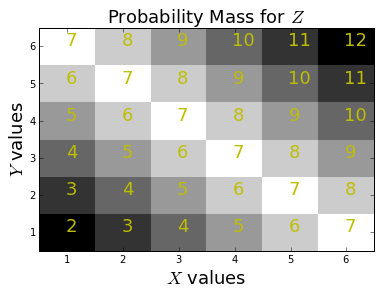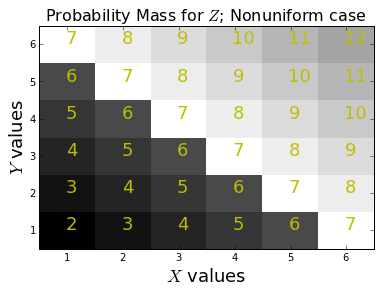## Wednesday, November 14, 2012

### Conditional Expectation and Mean Squared Errors

There are lots of statistics in statistical signal processing, but to use statistics effectively with signals, it helps to have a certain unifying perspective on both. To introduce these ideas, let's start with the powerful and intimate connection between least mean-squared-error (MSE) problems and conditional expectation that is sadly not emphasized in most courses.

Let's start with an example: suppose we have two fair six-sided die ($X$ and $Y$) and I want to measure the sum of the two variables as $Z=X+Y$. Further, let's suppose that given $Z$, I want the best estimate of $X$ in the mean-squared-sense. Thus, I want to minimize the following:

$$J(\alpha) = \sum ( x - \alpha z )^2 \mathbb{P}(x,z)$$

Here $\mathbb{P}$ encapsulates the density (i.e. mass) function for this problem. The idea is that when we have solved this problem, we will have a function of $Z$ that is going to be the minimum MSE estimate of $X$.

We can substitute in for $Z$ in $J$ and get:

$$J(\alpha) = \sum ( x - \alpha (x+y) )^2 \mathbb{P}(x,y)$$

Let's work out the steps in sympy in the following:

In :
import sympy
from sympy import stats, simplify, Rational, Integer,Eq
from sympy.stats import density, E
from sympy.abc import a

x=stats.Die('D1',6) # 1st six sided die
y=stats.Die('D2',6) # 2nd six sides die
z = x+y             # sum of 1st and 2nd die

J = stats.E((x - a*(x+y))**2)         # expectation
sol=sympy.solve(sympy.diff(J,a),a) # using calculus to minimize
print sol # solution is 1/2

1/2


This says that $z/2$ is the MSE estimate of $X$ given $Z$ which means geometrically ( interpreting the MSE as a squared distance weighted by the probability mass function) that $z/2$ is as close to $x$ as we are going to get for a given $z$.

Let's look at the same problem using the conditional expectation operator $\mathbb{E}(\cdot|z)$ and apply it to our definition of $Z$, then

$$\mathbb{E}(z|z) = \mathbb{E}(x+y|z) = \mathbb{E}(x|z) + \mathbb{E}(y|z) =z$$

where we've used the linearity of the expectation. Now, since by the symmetry of the problem, we have

$$\mathbb{E}(x|z) = \mathbb{E}(y|z)$$

we can plug this in and solve

$$2 \mathbb{E}(x|z) =z$$

which gives

$$\mathbb{E}(x|z) =\frac{z}{2}$$

which is suspiciously equal to the MSE estimate we just found. This is not an accident! The proof of this is not hard, but let's look at some pictures first

In :
fig, ax = subplots()
v = arange(1,7) + arange(1,7)[:,None]
foo=lambda i: density(z)[Integer(i)].evalf() # some tweaks to get a float out
Zmass=array(map(foo,v.flat),dtype=float32).reshape(6,6)

ax.pcolor(arange(1,8),arange(1,8),Zmass,cmap=cm.gray)
ax.set_xticks([(i+0.5) for i in range(1,7)])
ax.set_xticklabels([str(i) for i in range(1,7)])
ax.set_yticks([(i+0.5) for i in range(1,7)])
ax.set_yticklabels([str(i) for i in range(1,7)])
for i in range(1,7):
for j in range(1,7):
ax.text(i+.5,j+.5,str(i+j),fontsize=18,color='y')
ax.set_title(r'Probability Mass for $Z$',fontsize=18)
ax.set_xlabel('$X$ values',fontsize=18)
ax.set_ylabel('$Y$ values',fontsize=18);The figure shows the values of $Z$ in yellow with the corresponding values for $X$ and $Y$ on the axes. Suppose $z=2$, then the closest $X$ to this is $X=1$, which is what $\mathbb{E}(x|z)=z/2=1$ gives. What's more interesting is what happens when $Z=7$? In this case, this value is spread out along the $X$ axis so if $X=1$, then $Z$ is 6 units away, if $X=2$, then $Z$ is 5 units away and so on.

Now, back to the original question, if we had $Z=7$ and I wanted to get as close as I could to this using $X$, then why not choose $X=6$ which is only one unit away from $Z$? The problem with doing that is $X=6$ only occurs 1/6 of the time, so I'm not likely to get it right the other 5/6 of the time. So, 1/6 of the time I'm one unit away but 5/6 of the time I'm much more than one unit away. This means that the MSE score is going to be worse. Since each value of $X$ from 1 to 6 is equally likely, to play it safe, I'm going to choose $7/2$ as my estimate, which is what the conditional expectation suggests.

We can check this claim with samples using sympy below:

In :
#generate samples conditioned on z=7
samples_z7 = lambda : stats.sample(x, sympy.Eq(z,7)) # Eq constrains Z
mn= mean([(6-samples_z7())**2 for i in range(100)]) #using 6 as an estimate
mn0= mean([(7/2.-samples_z7())**2 for i in range(100)]) #7/2 is the MSE estimate
print 'MSE=%3.2f using 6 vs MSE=%3.2f using 7/2 ' % (mn,mn0)

MSE=9.99 using 6 vs MSE=2.97 using 7/2


Please run the above code repeatedly until you have convinced yourself that the $\mathbb{E}(x|z)$ gives the lower MSE every time.

To push this reasoning, let's consider the case where the die is so biased so that the outcome of 6 is ten times more probable than any of the other outcomes as in the following:

In :
# here 6 is ten times more probable than any other outcome
x=stats.FiniteRV('D3',{1:Rational(1,15), 2:Rational(1,15), 3: Rational(1,15),
4:Rational(1,15), 5:Rational(1,15), 6: Rational(2,3)})
z = x + y

# now re-create the plot
fig, ax = subplots()
foo=lambda i: density(z)[Integer(i)].evalf() # some tweaks to get a float out
Zmass=array(map(foo,v.flat),dtype=float32).reshape(6,6)

ax.pcolor(arange(1,8),arange(1,8),Zmass,cmap=cm.gray)
ax.set_xticks([(i+0.5) for i in range(1,7)])
ax.set_xticklabels([str(i) for i in range(1,7)])
ax.set_yticks([(i+0.5) for i in range(1,7)])
ax.set_yticklabels([str(i) for i in range(1,7)])
for i in range(1,7):
for j in range(1,7):
ax.text(i+.5,j+.5,str(i+j),fontsize=18,color='y')
ax.set_title(r'Probability Mass for $Z$; Nonuniform case',fontsize=16)
ax.set_xlabel('$X$ values',fontsize=18)
ax.set_ylabel('$Y$ values',fontsize=18);As compared with the first figure, the probability mass has been shifted away from the smaller numbers. Let's see what the conditional expectation says about how we can estimate $X$ from $Z$.

In :
E(x, Eq(z,7)) # conditional expectation E(x|z=7)

Out :
5

Now that we have $\mathbb{E}(x|z=7) = 5$, we can generate samples as before and see if this gives the minimum MSE.

In :
#generate samples conditioned on z=7
samples_z7 = lambda : stats.sample(x, Eq(z,7)) # Eq constrains Z
mn= mean([(6-samples_z7())**2 for i in range(100)]) #using 6 as an estimate
mn0= mean([(5-samples_z7())**2 for i in range(100)]) #7/2 is the MSE estimate
print 'MSE=%3.2f using 6 vs MSE=%3.2f using 5 ' % (mn,mn0)

MSE=3.27 using 6 vs MSE=2.92 using 5


### Summary

Using a simple example, we have emphasized the connection between minimum mean squared error problems and conditional expectation. Next, we'll continue revealing the true power of the conditional expectation as we continue to develop a corresponding geometric intuition.

As usual, the corresponding ipython notebook for this post is available for download here.

Comments and corrections welcome!

#### Post a Comment

Note: Only a member of this blog may post a comment.